# Quiz 15: Lean Accounting and Productivity Measurement

1.Calculation of total time to produce batch of 20 units using A Company traditional department structure is as follows: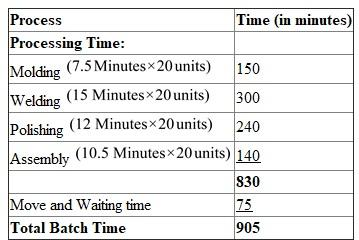Explanation: 1. We can calculate the processing time by multiplying time (minutes) with produce a batch of units (20units). Then we get the processing time of all departments. Then add all the processing times of all departments. 2. Calculate Move and waiting time. Processing time subtracts with move and waiting time then we get the Total time. 2.Calculation showing time saved over traditional manufacturing is as follows: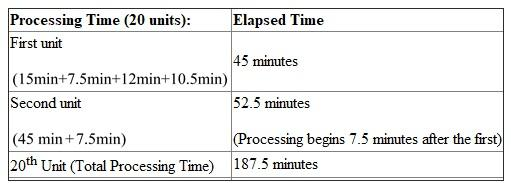Time saved over traditional manufacturing is 717.5 minutes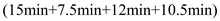If the cell is processing continuously, then a unit is produced every 15 minutes after the start-up unit. Thus, the Production rate is 4 units per hour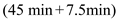The Bottleneck process controls the production rate. 3.The processing times of molding, welding, and assembly are all reduces to six minutes each then, Now the longest per unit processing time is 12 minutes. So, the Production rate will be 5 units per hour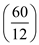. T ime taken to produce batch of 20 units will be 240 minutes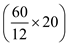.
1.Calculation of the unit cost is as follows: Given information: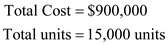Substitute and calculate unit cost:The Cost is very accurate as the value-stream cost and total units shipped apply to only one product and thus, the total cost also belongs to that product. 2.Calculation of the unit cost is as follows: Given information:Substitute and calculate unit cost:Explanation: Each unit of Model A and B will have the same cost i.e. \$60 per unit. Using units shipped instead of units produced for the unit calculation motivates managers to reduce inventories. 3.The unit materials cost is calculated separately as follows:Model A is responsible for 40%, Remaining 60%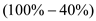B is responsible for material cost.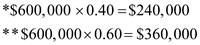Calculate unit material cost for model A by dividing Totals with units. Then we get the Unit material cost for Model A. Calculation of Average unit conversion cost:Calculation of Unit Cost: